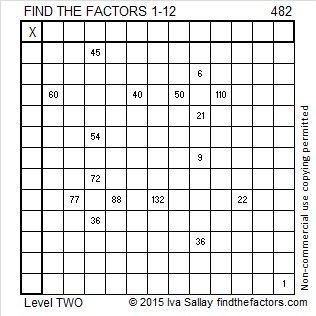# 482 and Level 2

Can you determine the greatest common factor of the three numbers in this Pythagorean triple: 240-418-482? Hint: it is one of the factors of 482 listed below the puzzle.Print the puzzles or type the solution on this excel file: 12 Factors 2015-05-04

—————————————————————————————————

• 482 is a composite number.
• Prime factorization: 482 = 2 x 241
• The exponents in the prime factorization are 1 and 1. Adding one to each and multiplying we get (1 + 1)(1 + 1) = 2 x 2 = 4. Therefore 482 has exactly 4 factors.
• Factors of 482: 1, 2, 241, 482
• Factor pairs: 482 = 1 x 482 or 2 x 241
• 482 has no square factors that allow its square root to be simplified. √482 ≈ 21.954498—————————————————————————————————

## 2 thoughts on “482 and Level 2”

1.nikirath

2.ivasallay